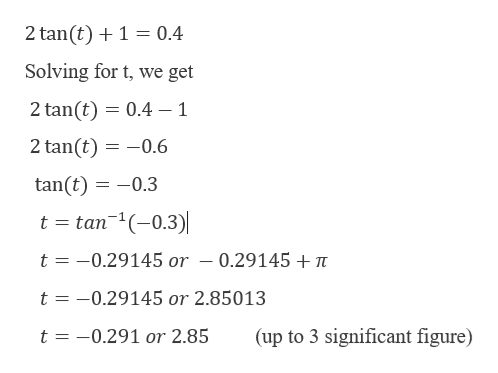# Solve the following equation over the indicated interval. A calculator is required. Calculated answers should be to at least 3 sig figs.  2tan t + 1= 0.4 where 0< t<2pi

Question
1 views

Solve the following equation over the indicated interval. A calculator is required. Calculated answers should be to at least 3 sig figs.

2tan t + 1= 0.4 where 0< t<2pi

check_circle

Step 1

Given,

Step 2

Now,

...help_outlineImage Transcriptionclose2 tan (t)1 0.4 Solving for t, we get 2 tan(t) 0.4 - 1 2 tan(t) 0.6 tan(t) -0.3 t tan1(0.3) t -0.29145 or - 0.29145 + t t -0.29145 or 2.85013 t -0.291 or 2.85 (up to 3 significant figure) fullscreen

### Want to see the full answer?

See Solution

#### Want to see this answer and more?

Solutions are written by subject experts who are available 24/7. Questions are typically answered within 1 hour.*

See Solution
*Response times may vary by subject and question.
Tagged in

### Trigonometric Ratios